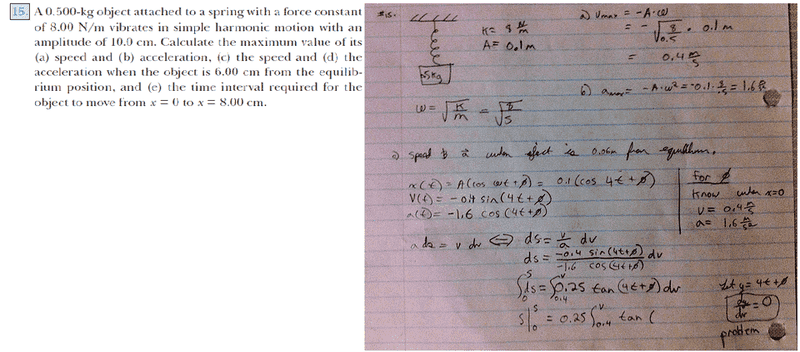# Simple harmonic motion problem, finding velocity as a function of position

## Homework StatementI need help finding velocity as a function of position, the t in the argument of tan, causes a problem for me when integrating. can someone help, maybe my approach is completly off, i am trying to fing the unknown phi, knowing the velocity and acceletation when position is 0.

matt

rock.freak667
Homework Helper
So you have x= Acos(ωt+φ), you should know that when x=0,t=0 meaning that you can get the value of φ easily.

As for the integration, once you get φ, it would be easier to start with

a=-ω2x

v(dv/dx)=-ω2x

v dv = -ω2x dx

∫v dv = -ω2∫ x dx.

So you have x= Acos(ωt+φ), you should know that when x=0,t=0 meaning that you can get the value of φ easily.

As for the integration, once you get φ, it would be easier to start with

a=-ω2x

v(dv/dx)=-ω2x

v dv = -ω2x dx

∫v dv = -ω2∫ x dx.

the problem doesnt specify that when x = 0, t =0, how can i make such an assumption?

rock.freak667
Homework Helper
the problem doesnt specify that when x = 0, t =0, how can i make such an assumption?

Yes you are right, I thought your expression for x had the sine term in it, sorry about that.

no problem, i think this boils down to integrating that above statement, im sorry for the poor image quality, my scanner broke.
somehow i need phi, im not quite sure how to obtain it. i think parts a and b we intentional to get me thinking in the way of integration.

nvn
Homework Helper
Matt1234: You can see, at t = t1 = 0, x1 = 0.10 m. In other words, you need to fully deflect the mass, then release it, in order for it to vibrate. Therefore, at t1 = 0, we have,

x1 = 0.10 m = (0.10 m)*cos(4*t1 + phi)
cos(4*t1 + phi) = 1
4*t1 + phi = acos(1)
phi = acos(1) - 4*t1
phi = 0 - 4*0

Therefore, we have,

x(t) = A*cos(omega*t + phi)
x(t) = A*cos(omega*t + 0)
x(t) = A*cos(omega*t)​

Likewise, v(t) = -A*omega*sin(omega*t).

(c) Let x(t2) = 0.06 m. Therefore,

x(t2) = A*cos(omega*t2)
0.06 = 0.10*cos(4*t2)​

Hint 1: Can you solve for t2? After that, can you compute v(t2)?

(d) Hint 2: Can you compute a(t2)?

(e) Hint 3: Let x(t3) = 0 m. Can you solve for t3? Hint 4: Let x(t4) = -0.08 m. Can you solve for t4?

Try again. Also, please do not post wide images directly to the forum page. Just post a text link to wide images.